# GSEB Solutions Class 10 Maths Chapter 3 Pair of Linear Equations in Two Variables Ex 3.2

Gujarat Board GSEB Solutions Class 10 Maths Chapter 3 Pair of Linear Equations in Two Variables Ex 3.2 Textbook Questions and Answers.

## Gujarat Board Textbook Solutions Class 10 Maths Chapter 3 Pair of Linear Equations in Two Variables Ex 3.2

Question 1.
Form the pair of linear equations in the following problems, and find their solutions graphically.

1. 10 students of Class X took part in a Mathematics quiz. If the number of girls is 4 more than the number of boys, find the number of boys and girls who took part in the quiz.
2. 5 pencils and 7 pens together cost ₹ 50, whereas 7 pencils and 5 pens together cost ₹ 46. Find the cost of one pencil and that of one pen.

Solution:
1. Let the number of boys and girls who took part in the quiz be x and y respectively. Then, the pair of linear equations formed is x + y – 10          …(1)
and y – x + 4             …(2)
Let us draw the graphs of equations (1) and (2) by finding two solutions for each of the equations. These two solutions of the equations (1) and (2) are given below in table 1 and table 2 respectively.
For equation (1)
x + y – 10
⇒ y = 10 – x
Table 1For equation (2)
y = x + 4
Table 2We plot the points A(6, 4) and B(4, 6) on a graph paper and join these points to form the line AB representing the equation (1) as shown in the paper. Also, we plot the points C(0, 4) and D(l, 5) on the same graph paper and join these points to form the line CD representing the equation (2) as shown in the same figure.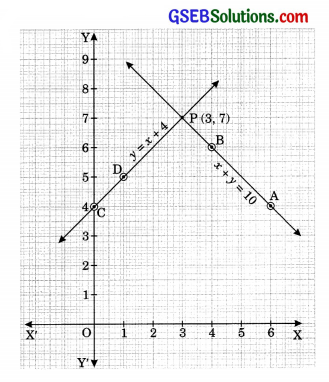In the figure, we observe that the two lines intersect at the point P(3, 7). So x = 3 and y = 7 is the required solution of the pair of linear equations formed, i.e. the number of boys and girls who took part in the quiz is 3 and 7 respectively.

Verification:
Substitution x = 3 and y = 7 in (1) and (2), we find that both the equa-tions are satisfied as shown below:
x + y = 3 + 7 = 10
x + 4 = 3 + 4 = 7 = y
This verifies the solutions.2. Let the cost of one pencil and a pen be ₹ x and ₹ y respectively. Then the pair of linear equations formed is
5x + 7y = 50        …(1)
and 7x + 5y = 46  …(2)
Let us draw the graphs of the equations (1) and (2) by finding two solutions for each of the equation. These two solutions of the equations (1) and (2) are given below in Table 1 and Table 2 respectively.
For equation (1)
5x + 7y = 50
⇒ 7y = 50 – 5x
⇒ y = $$\frac{50-5 x}{7}$$
Table 1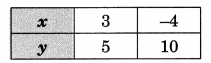For equation (2)
7x + 5y = 46
⇒ 5y = 46 – 7x
46-7x
⇒ y = $$\frac{46-7 x}{5}$$Table 2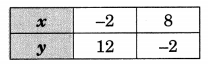We plot the points A(3, 5) and B(-4, 10) on a graph paper and join these points to form the line AB representing the equation (1) as shown in the figure. Also, we plot the points C(-2, 12) and D(8, -2) on the same graph paper and join these points to form the line CD representing the equation (2) as shown in the same figure.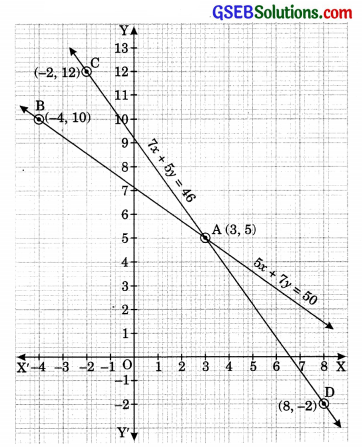In the figure, we observe that the two lines intersect at the point A(3, 5). So x = 3 and y = 5 is the required solution of the pair of linear equations formed, i.e., the cost of one pencil is ₹ 3 and that of a pen is ₹ 5.
Verification:
Substituting x = 3 and y = 5 in (1) and (2), we find that both the equations are satisfied as shown below:
5x + 7y = 5(3) + 7(5) = 15 + 35 = 50
7x + 5y = 7(3) + 5(5) = 21 + 25 = 46
This verifies the solution.Question 2.
On comparing the ratios $$\frac{a_{1}}{a_{2}}, \frac{b_{1}}{b_{2}}$$ and $$\frac{c_{1}}{c_{2}}$$, find out whether the lines representing the following pairs of linear equations intersect at a point, parallel or coincident:

1. 5x – 4y + 8 = 0, 7x + 6y – 9 = 0
2. 9x + 3y + 12 = 0, 18x + 6y + 24 = 0
3. 6x – 3y + 10 = 0, 2x – y + 9 = 0

Solution:
1. 5x – 4y + 8 = 0
7x + 6y – 9 = 0
Here, a1 = 5, b1 = -4, c1 = 8
a2 = 7, b2 = 6, C2 = -9
We see that $$\frac{a_{1}}{a_{2}} \neq \frac{b_{1}}{b_{2}}$$
Hence, the lines representing the given pair of linear equations intersect at a point.

2. 9x + 3y + 12 = 0
18x + 6y + 24 = 0
Here, a1 = 9, b1 = 3, c1 = 12
a2 = 18, b2 = 6, c2 = 24
We see that $$\frac{a_{1}}{a_{2}}=\frac{b_{1}}{b_{2}}=\frac{c_{1}}{c_{2}}$$
Hence, the lines representing the given pair of linear equations are coincident.3. 6x – 3y + 10 = 0
2x – y + 9 = 0
Here, a1 = 6, b1 = -3, c1 = 10
a2 = 2, b2 = -1, c2 = 9
We see that $$\frac{a_{1}}{a_{2}}=\frac{b_{1}}{b_{2}} \neq \frac{c_{1}}{c_{2}}$$
Hence, the lines representing the given pair of linear equations are parallel.

Question 3.
On comparing the ratios $$\frac{a_{1}}{a_{2}}, \frac{b_{1}}{b_{2}} \text { and } \frac{c_{1}}{c_{2}}$$, find out whether the following pair of linear equations are consistent, or inconsistent.

1. 3x + 2y = 5; 2x – 3y = 7
2. 2x – 3y = 8; 4x – 5y = 9
3. $$\frac{3}{2}$$x + $$\frac{5}{3}$$y = 7; 9x – 10y = 14
4. 5x – 3y = 11; -10x + 6y = -22
5. $$\frac{4}{3}$$x + 2y = 8; 2x + 3y = 12

Solution:
1. 3x + 2y = 5; 2x – 3y = 7
Here, a1 = 3, b1 = 2, c1 = -5
a2 = 2, b2 = -3, c2 = -7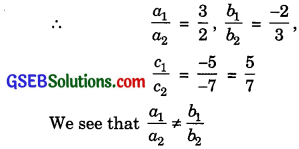Hence, the given lines are intersecting. So, the given pair of linear equations has exactly one (unique) solution and therefore it is consistent.

2. 2x – 3y = 8; 4x – 6y = 9
Here, a1 = 2, b1 = -3, c1 = -8
a2 = 4, b2 = -6, c2 = -9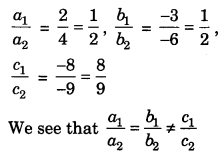Hence, the given lines are parallel. So, the given pair of linear equations has no solution and therefore it is inconsistent.3. $$\frac{3}{2}$$x + $$\frac{5}{3}$$y = 7; 9x – 10y = 14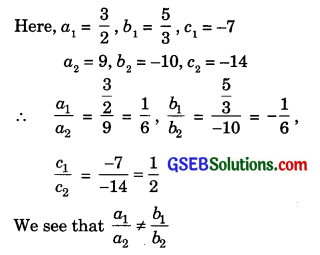Hence, the given lines are intersecting. So, the given pair of linear equations has exactly one (unique) solution and therefore it is consistent.

4. 5x – 3y = 11; -10x + 6y = -22
Here, a1 = 5, b1 = -3, c1 = -11
a2 = -10, b2 = 6, c2 = 22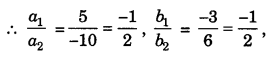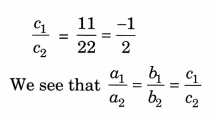Hence, the given lines are coincident. So, the given pair of linear equations has infinitely many solutions and therefore it is consistent (dependent).5. $$\frac{4}{3}$$x + 2y = 8; 2x + 3y = 12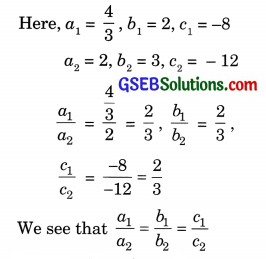Hence, the given lines are coincident. So, the given pair of linear equations has infinitely many solutions and therefore it is consistent (dependent).

Question 4.
Which of the following pairs of linear equations are consistent/inconsistent? If consistent, obtain the solution graphically:

1. x + y = 5, 2x + 2y = 10
2. x – y – 8, 3x – 3y = 16
3. 2x + y – 6 = 0, 4x – 2y – 4 = 0
4. 2x – 2y – 2 = 0, 4x – 4y – 5 = 0

Solution:
1. x + y = 5 …(1)
2x + 2y = 10 …(2)
Here, a1 = 1, b1 = 1, c1 = -5
a2 = -2, b2 = 2, c2 = -10
We see that $$\frac{a_{1}}{a_{2}}=\frac{b_{1}}{b_{2}}=\frac{c_{1}}{c_{2}}$$
Hence, the lines represented by the equations (1) and (2) are coincident. Therefore, equations (1) and (2) have infinitely many common solutions, i.e., the given pair of linear equations is consistent.For graphical representation, we draw the graphs of the equations (1) and (2) by finding two solutions for each of the equations. These two solutions of the equations (1) and (2) are given below in table 1 and table 2 respectively.
For equation (1)
⇒ x + y = -5
y = 5 – x
Table 1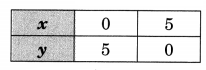For equation (2)
2x + 2y = 10
⇒ 2y = 10 – 2x
⇒ y = $$\frac{10-2 x}{2}$$
⇒ y = 5 – x
Table 2We plot the points A(0, 5) and B(5, 0) on a graph paper and join these points to form the line AB representing the equation (1) and points C(l, 4) and D(2, 3) on the same graph paper and join these points to form the line CD representing the equation (2) as shown in the same figure.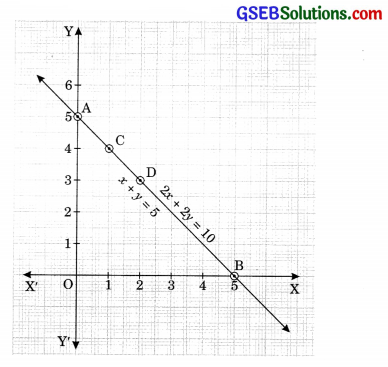In the figure we observe that the two lines AB and CD are coincident.2. x – y = 8 …(1)
3x – 3y = 16 …(2)
Here, a1 = 1, b1 = -1, c1 = – 8
a2 = 3, b2 = -3, c2 = -16
We see that $$\frac{a_{1}}{a_{2}}=\frac{b_{1}}{b_{2}} \neq \frac{c_{1}}{c_{2}}$$
Hence, the lines represented by the equations (1) and (2) are parallel. Therefore, equations (1) and (2) have no solution, i.e., the given pair of linear equation is inconsistent.

3. 2x + y – 6 = 0 …(1)
4x – 2y – 4 = 0 …(2)
Here, a1 = 2, b1 = 1, c1 = -6
a2 = 4, b2 = -2, c2 = -4
We see that $$\frac{a_{1}}{a_{2}} \neq \frac{b_{1}}{b_{2}}$$
Hence, the lines represented by the equations (1) and (2) are intersecting. Therefore, equations (1) and (2) have exactly one (unique) solution i.e., the given pair of linear equation is consistent.

Graphical representation:
We draw the graphs of the equations (1) and (2) by finding two solutions for each of the equations. These two solutions of the equations (1) and (2) are given below in table 1 and table 2 respectively.
For equation (1)
2x + y – 6 = 0
⇒ y = -2x + 6
Table 1For equation (2)
4x – 2y – 4 = 0
⇒ 2y = 4x – 4
⇒ y = $$\frac{4 x-4}{2}$$
⇒ y = 2x – 2
Table 2We plot the points A(0, -6) and B(3, 0) on a graph paper and join these points to form the line AB representing the equation (1) as shown in the figure. Also we plot the points C(0, -2) and D(l, 0) on the same graph paper and join these points to form the line CD representing the equation (2) as shown in the same figure.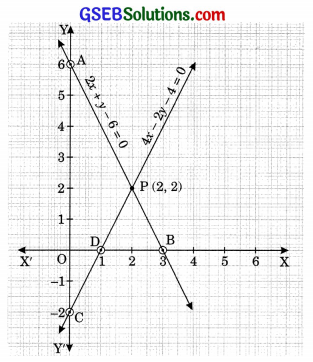In the figure we observe that the two lines intersect at the point P(2, 2). So x – 2 and y – 2 is the required unique solution of the pair of linear equations formed.

Verification:
Substituting x = 2 and y = 2 in (1) and (2), we find that both the equations are satisfied as shown below:
2x – 2y – 6 = 2(2) + 2 – 6 = 0
4x – 2y – 4 = 4(2) – 2(2) – 4 = 0
This verifies the solution.4. 2x – 2y – 2 = 0 …(1)
4x – 4y – 5 = 0 …(2)
Here, a1 = 2, b1 = -2, c1 = -2
a2 = 4, b2 = -4, c2 = -5
We see that $$\frac{a_{1}}{a_{2}}=\frac{b_{1}}{b_{2}} \neq \frac{c_{1}}{c_{2}}$$
Hence, the lines represented by the equations (1) and (2) are parallel. Therefore, equations (1) and (2) have no solution, i.e., the given pair of linear equation is inconsistent.

Question 5.
Half the perimeter of a rectangular garden, whose length is 4 m more than its width is 36 m. Find the dimensions of the garden.
Solution:
Let the dimensions (i.e., length and width) of the garden be x m and y m respectively. Then,
x = y + 4
⇒ x – y = 4 …(1)
and $$\frac{1}{2}$$ (2x + 2y) = 36
⇒ x +y = 36 …(2)Let us draw the graphs of equations (1) and (2) by finding two solutions for each of the equations.
These two solutions of the equations (1) and (2) are given below in table 1 and table 2 respectively.
For equation (1)
x – y = 4
⇒ y = x – 4
Table 1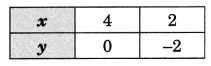For equation (2)
x + y = 36
y = 36 – x
Table 2We plot the points A(4, 0) and B(2, -2) on a graph paper and join these points to form the line AB representing the equation (1) as shown in the figure. Also, we plot the points C(20, 16), D(16, 20) on the same graph paper and join these points to form the line CD representing the equation (2) as shown in the same figure.

In the figure we observe that the two lines intersect at the point C(20, 16). So x = 20, y = 16 is the required solution of the pair of linear equations formed, i.e., the dimensions of the garden are 20 m and 16 m.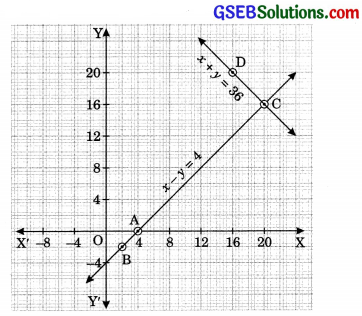Verification:
Substituting x = 20 and y = 16 in (1) and (2), we find that both the equations are satisfied as shown below:
20 – 16 = 4
20 + 16 = 36
This verifies the solution.Question 6.
Given the linear equation 2x + 3y – 8 = 0, write another linear equation in two variables such that the geometrical representation of the pair so formed is:

1. Intersecting lines
2. Parallel lines
3. Coincident lines

Solution:
1. 2x + 3y – 8 = 0
3x + 2y – 7 = 0

2. 2x + 3y – 8 = 0
2x + 3y – 12 = 0

3. 2x + 3y – 8 = 0
4x + 6y – 16 = 0Question 7.
Draw the graphs of the equations x – y + 1 = 0 and 3x + 2y – 12 = 0. Determine the coordinates of the vertices of the triangle formed by these lines and the x-axis, and shade the triangular region.
Solution:
The given equations are
x – y + 1 = 0 …(1)
3x + 2y – 12 = 0 …(2)
Let us draw the graphs of equations (1) and (2) by finding two solutions for each of the equation. These two solutions of the equation (1) and (2) are given below in table 1 and table 2 respectively.
For equation (1)
x – y + 1 = 0
⇒ y = x + 1
Table 1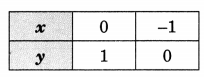For equation (2)
3x + 2y – 12 = 0
⇒ 2y = 12 – 3x
y $$=\frac{12-3 x}{2}$$
Table 2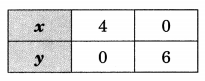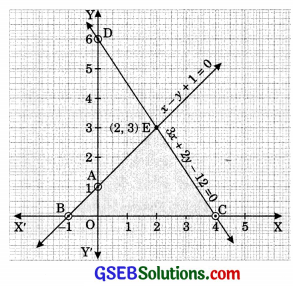We plot the points A(0, 1) and B(-1, 0) on a graph paper and join these points to form the line AB representing the equation (1) as shown in the figure. Also, we plot the points C(4, 0) and D(0, 6) on the same graph paper and join these points to form the line CD representing the equation (2) as shown in the same figure.

In the figure we observe that the coordinates of the vertices of the triangle formed by these given lines the x-axis are E(2, 3), B(-1, 0) and C(4, 0).